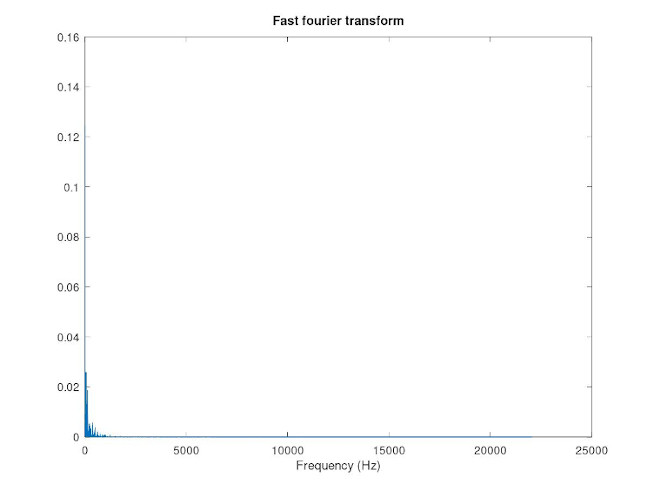## October – Fast Fourier Transform in GNU Octave

Fast fourier transform (FFT) is an algorithm that computes the discrete Fourier transform.

FFT is especially handy in real-time digital signal processing. Digital computers are working on discrete data, thus a input signal is always sampled with some sampling rate(Hz). By the Nyquist-Shannon sampling theorem, sampling captures frequencies under.

Octave calculates the FFT of a discrete signal. In the following code, we calculate the normalized FFT and plot the frequency spectrum w.r.t. the frequency. Signal- and sample time vectors are given as input.

```##Calculates the FFT and plots the frequency spectrum of a signal. Sampletimes have to start at time 0.

function fftvector = plotfft(signal, sampletimes)
N = length(sampletimes); #Signal length.
Fs = (N - 1)/(sampletimes(N)) #Sampling rate. We have N - 1 "hops" in sampletimes(N) seconds.
FFT =  fft(signal);
if(mod(N,2) == 0) #Check if signal length is odd or even.
FFT = 2*FFT(1 : N/2)/N;
f = Fs*(0 : (N - 1)/2)/(N - 1);
else
FFT = 2*FFT(1 : (N - 1)/2)/N;
f = Fs*(0 : (N - 2)/2)/(N - 1);
end
fftvector = [f; FFT];

figure(1)
plot(f, abs(FFT));
title('Fast fourier transform')
xlabel('Frequency (Hz)')
print  plot.jpg
end
```

The following figure shows my track Tappimarssi in the frequency domain. The track was imported to Octave by

```[signal, fs] = audioread('Tappimarssi.wav');
sampletimes = 0 : 1/fs : 1/fs*(length(signal) - 1);
```